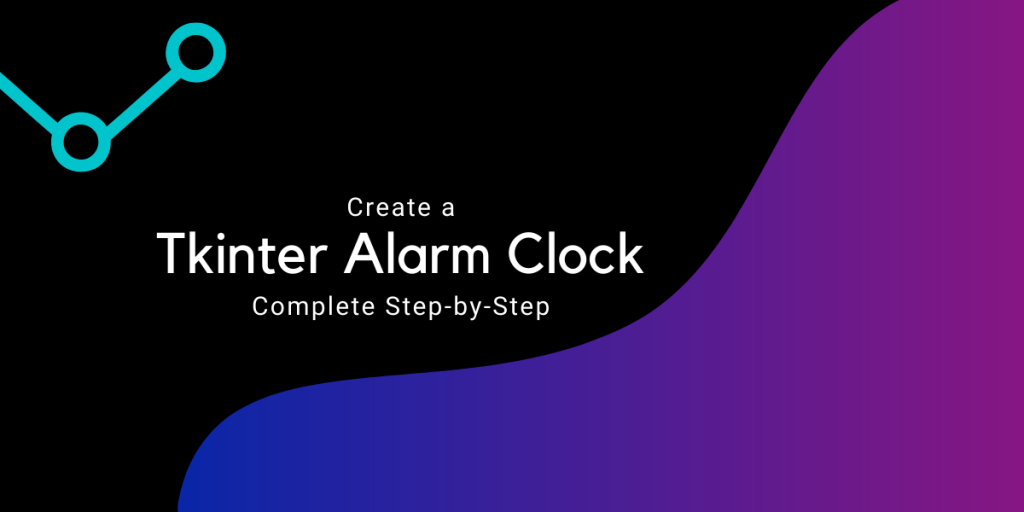# Tkinter Alarm Clock – A Step-By-Step GuideHello there! Today in this tutorial, we will be developing a basic Python Tkinter Alarm Clock.

There is no wonder that an alarm clock is always useful to warn us while we sleep, take a brief nap, or to remind us about the job, we always get ignorant about.

Recommended read: Python Tkinter GUI Calculator

## Introduction to the Project

The project makes use of some python libraries namely, datetime and Tkinter.

The project makes use of the current date and time along with a feature to set an alarm according to the current date and time found.

## Building the Tkinter Alarm Clock

Let’s not waste anymore time and start off building the project now!

### 1. Importing required modules

Before building any project, the first step is importing all the necessary libraries and modules that we require for the project.

```from tkinter import *
import datetime
import time
import winsound
```

Let’s know about each module we have just imported:

1. Tkinter module: Helps us to create a window for the user to use the application
2. datetime and time modules: Help us to handle dates and time and manipulate them when needed.
3. winsound module: Helpful to generate sounds for our alarm clock.

### 2. Creating a function for the alarm

The next step involves creating functions for the alarm clock. Let’s look at the code for the same first.

```def Alarm(set_alarm_timer):
while True:
time.sleep(1)
actual_time = datetime.datetime.now()
cur_time = actual_time.strftime("%H:%M:%S")
cur_date = actual_time.strftime("%d/%m/%Y")
msg="Current Time: "+str(cur_time)
print(msg)
if cur_time == set_alarm_timer:
winsound.PlaySound("Music.wav",winsound.SND_ASYNC)
break

def get_alarm_time():
alarm_set_time = f"{hour.get()}:{min.get()}:{sec.get()}"
Alarm(alarm_set_time)
```

The function named `Alarm` handles the main functionality of the application. The function takes the alarm time the user sets in the entry boxes of the window as an argument.

`sleep` function stops the execution of the program until it gets the time values entered by the user.

Then we get the current date and time using the `datetime.now` function and store the time and date into separate variables with the help of `strftime` function.

The the program checks when the current time matches the alarm time set by the user. When the condition is true then the sound is played using the `winsound` module or else the timer continues.

A new function is defined to get the input from the user entry boxes and pass it to the previous function.

### 3. Creating the Tkinter Window

The final step is to create the main window of the application with all the widgets and features defined. The code for the same is shown below.

```window = Tk()
window.title("Alarm Clock")
window.geometry("400x160")
window.config(bg="#922B21")
window.resizable(width=False,height=False)

time_format=Label(window, text= "Remember to set time in 24 hour format!", fg="white",bg="#922B21",font=("Arial",15)).place(x=20,y=120)

addTime = Label(window,text = "Hour     Min     Sec",font=60,fg="white",bg="black").place(x = 210)
setYourAlarm = Label(window,text = "Set Time for Alarm: ",fg="white",bg="#922B21",relief = "solid",font=("Helevetica",15,"bold")).place(x=10, y=40)

hour = StringVar()
min = StringVar()
sec = StringVar()

hourTime= Entry(window,textvariable = hour,bg = "#48C9B0",width = 4,font=(20)).place(x=210,y=40)
minTime= Entry(window,textvariable = min,bg = "#48C9B0",width = 4,font=(20)).place(x=270,y=40)
secTime = Entry(window,textvariable = sec,bg = "#48C9B0",width = 4,font=(20)).place(x=330,y=40)

submit = Button(window,text = "Set Your Alarm",fg="Black",bg="#D4AC0D",width = 15,command = get_alarm_time,font=(20)).place(x =100,y=80)

window.mainloop()
```

## Complete Code for Tkinter Alarm Clock

```from tkinter import *
import datetime
import time
import winsound

def Alarm(set_alarm_timer):
while True:
time.sleep(1)
actual_time = datetime.datetime.now()
cur_time = actual_time.strftime("%H:%M:%S")
cur_date = actual_time.strftime("%d/%m/%Y")
msg="Current Time: "+str(cur_time)
print(msg)
if cur_time == set_alarm_timer:
winsound.PlaySound("Music.wav",winsound.SND_ASYNC)
break

def get_alarm_time():
alarm_set_time = f"{hour.get()}:{min.get()}:{sec.get()}"
Alarm(alarm_set_time)

window = Tk()
window.title("Alarm Clock")
window.geometry("400x160")
window.config(bg="#922B21")
window.resizable(width=False,height=False)

time_format=Label(window, text= "Remember to set time in 24 hour format!", fg="white",bg="#922B21",font=("Arial",15)).place(x=20,y=120)
addTime = Label(window,text = "Hour     Min     Sec",font=60,fg="white",bg="black").place(x = 210)
setYourAlarm = Label(window,text = "Set Time for Alarm: ",fg="white",bg="#922B21",relief = "solid",font=("Helevetica",15,"bold")).place(x=10, y=40)

hour = StringVar()
min = StringVar()
sec = StringVar()

hourTime= Entry(window,textvariable = hour,bg = "#48C9B0",width = 4,font=(20)).place(x=210,y=40)
minTime= Entry(window,textvariable = min,bg = "#48C9B0",width = 4,font=(20)).place(x=270,y=40)
secTime = Entry(window,textvariable = sec,bg = "#48C9B0",width = 4,font=(20)).place(x=330,y=40)

submit = Button(window,text = "Set Your Alarm",fg="Black",bg="#D4AC0D",width = 15,command = get_alarm_time,font=(20)).place(x =100,y=80)

window.mainloop()
```

## Sample Output

The video below displays the working of the application. You can customize the window and variables according to your preferences.

## Conclusion

Congratulations! Today, we have successfully learned how to make an Alarm Clock using Tkinter module of Python. We also learned about extracting current date and time and playing sound at the particular instant of time.

Hope you liked it! Happy learning!